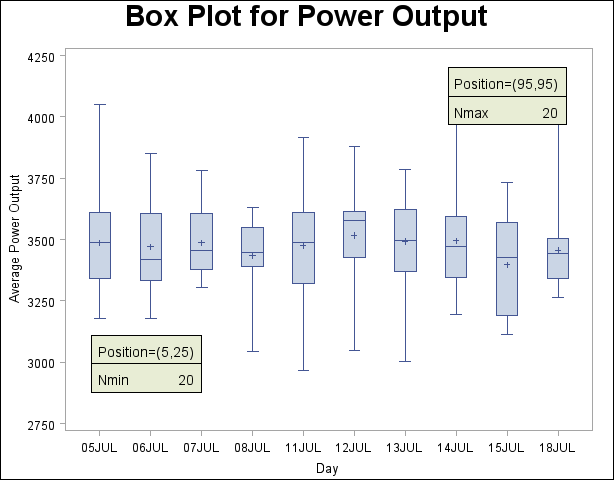The BOXPLOT Procedure
 Positioning Insets

This section provides details on three different methods of positioning INSET boxes by using the POSITION= option. With the POSITION= option, you can specify the following:

• compass points

• keywords for margin positions

• coordinates in data units or percent axis units

### Positioning the Inset Using Compass Points

You can specify the eight compass points (N, NE, E, SE, S, SW, W, and NW) as keywords for the POSITION= option. The default inset position is NW. The following statements create the display in Figure 24.11, which illustrates all eight compass positions:

```title 'Box Plot for Power Output';
proc boxplot data=Turbine;
plot KWatts*Day;
inset nobs / height=2.5 cfill=blank header='NW' pos=nw;
inset nobs / height=2.5 cfill=blank header='N ' pos=n ;
inset nobs / height=2.5 cfill=blank header='NE' pos=ne;
inset nobs / height=2.5 cfill=blank header='E ' pos=e ;
inset nobs / height=2.5 cfill=blank header='SE' pos=se;
inset nobs / height=2.5 cfill=blank header='S ' pos=s ;
inset nobs / height=2.5 cfill=blank header='SW' pos=sw;
inset nobs / height=2.5 cfill=blank header='W ' pos=w ;
run;
```

Figure 24.11 Insets Positioned Using Compass Points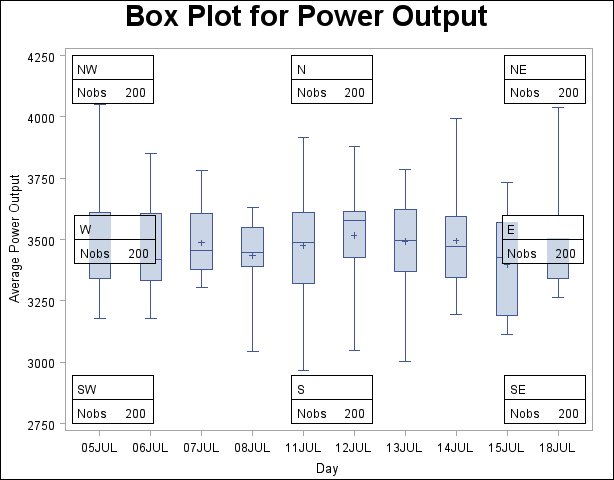### Positioning the Inset in the Margins

You can also use the INSET statement to position an inset in one of the four margins surrounding the plot area by using the margin keyword LM, RM, TM, or BM, as illustrated in Figure 24.12.

Figure 24.12 Positioning Insets in the Margins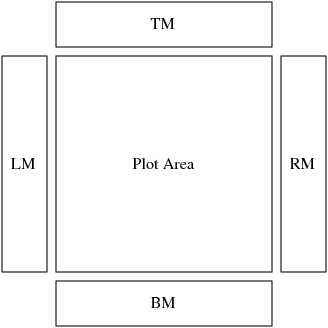For an example of an inset placed in the top margin, see Output 24.1.1. Margin positions are recommended for insets containing a large number of statistics. If you attempt to display a lengthy inset in the interior of the plot, it is likely that the inset will collide with the data display.

### Positioning the Inset Using Coordinates

You can also specify the position of an inset with coordinates by using the POSITION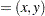option. You can specify coordinates in axis percent units (the default) or in axis data units.

#### Data Unit Coordinates

If you specify the DATA option immediately following the coordinates, the inset is positioned using axis data units. For example, the following statements place the bottom-left corner of the inset at 07JUL on the horizontal axis and 3950 on the vertical axis:

```title 'Box Plot for Power Output';
proc boxplot data=Turbine;
plot KWatts*Day;
inset nobs /
position = ('07JUL94'd, 3950) data;
run;
```

The box plot is displayed in Figure 24.13. By default, the specified coordinates determine the position of the bottom-left corner of the inset. You can change this reference point with the REFPOINT= option, as in the next example.

Figure 24.13 Inset Positioned Using Data Unit Coordinates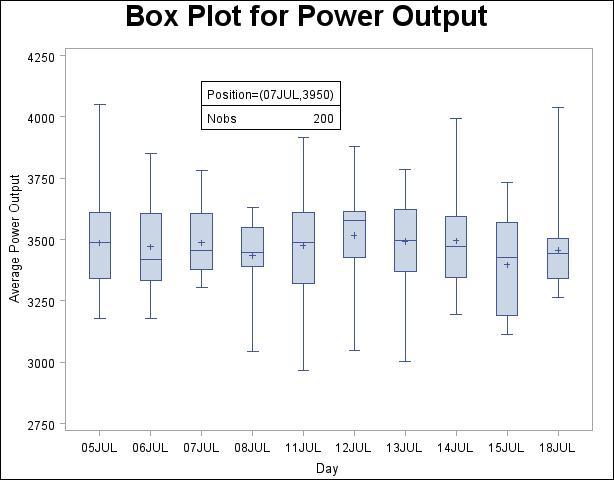#### Axis Percent Unit Coordinates

If you do not use the DATA option, the inset is positioned using axis percent units. The coordinates of the bottom-left corner of the display are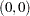, while the coordinates of the top-right corner are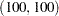. For example, the following statements create a box plot with two insets, both positioned using coordinates in axis percent units:

```title 'Box Plot for Power Output';
proc boxplot data=Turbine;
plot KWatts*Day;
inset nmin / position = (5,25)
height   = 3
cfill    = ywh
refpoint = tl;
inset nmax / position = (95,95)